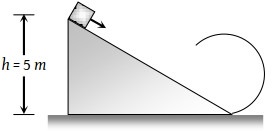You’ve reached the end of your free Videos limit.
#4 | Work Energy Theorem
(Physics) > Work,Energy and Power
Unable to watch the video, please try another server
Related Practice Questions :

A steel wire can withstand a load up to 2940 N. A load of 150 kg is suspended from a rigid support. The maximum angle through which the wire can be displaced from the mean position, so that the wire does not break when the load passes through the position of equilibrium, is (2008 E)

(1) 30$°$

(2) 60$°$

(3) 80$°$

(4) 85$°$

High Yielding Test Series + Question Bank - NEET 2020

Difficulty Level:

A ball is suspended by a thread of length l. What minimum horizontal velocity has to be imparted to the ball for it to reach the height of the suspension:

(1) gl

(2) 2 gl

(3) $\sqrt{gl}$

(4) $\sqrt{2gl}$

High Yielding Test Series + Question Bank - NEET 2020

Difficulty Level:

As per given figure to complete the circular loop what should be the radius if initial height is 5?(1) 4 m

(2) 3 m

(3) 2.5 m

(4) 2 m

High Yielding Test Series + Question Bank - NEET 2020

Difficulty Level:

A stone of mass 1 kg tied to a light inextensible string of length $L=\frac{10}{3}m$ is whirling in a circular path of radius L in a vertical plane. If the ratio of the maximum tension in the string to the minimum tension in the string is 4 and if g is taken to be 10 m/sec2, the speed of the stone at the highest point of the circle is

(1) 20 m/sec

(2) $10\sqrt{3}m/\mathrm{sec}$

(3) $5\sqrt{2}\text{\hspace{0.17em}}m/\mathrm{sec}$

(4) 10 m/sec

High Yielding Test Series + Question Bank - NEET 2020

Difficulty Level:

Which one of the following is not a conservative force

(1) Gravitational force

(2) Electrostatic force between two charges

(3) Magnetic force between two magnetic dipoles

(4) Frictional force